# python进阶—OpenCV之图像处理（三）

python opencv 图像处理第三篇。

# 图像直方图（Histograms）

## 直方图查找，绘制，分析

cv.calcHist此函数由三种实现，下面仅介绍第一种

• 函数原型：hist = cv.calcHist( images, channels, mask, histSize, ranges[, hist[, accumulate]] )
• images: 输入的图像或数组，它们的深度必须为CV_8U, CV_16U或CV_32F中的一类，尺寸必须相同。
• nimages: 输入数组个数，也就是第一个参数中存放了几张图像，有几个原数组。
• channels: 需要统计的通道dim，第一个数组通道从0到image.channels()-1，第二个数组从image.channels()到images.channels()+images.channels()-1，以后的数组以此类推
• hist: 输出的目标直方图，一个二维数组
• dims: 需要计算直方图的维度，必须是正数且并不大于CV_MAX_DIMS(在opencv中等于32)
• histSize: 每个维度的直方图尺寸的数组
• ranges: 每个维度中bin的取值范围
• uniform: 直方图是否均匀的标识符，有默认值true
• accumulate: 累积标识符，有默认值false,若为true，直方图再分配阶段不会清零。此功能主要是允许从多个阵列中计算单个直方图或者用于再特定的时间更新直方图.
此函数查找并计算直方图，但是并没有绘制直方图。
以下方式可以直接计算并绘制直方图
import numpy as np
import cv2 as cv
from matplotlib import pyplot as plt
plt.hist(img.ravel(),256,[0,256]); plt.show()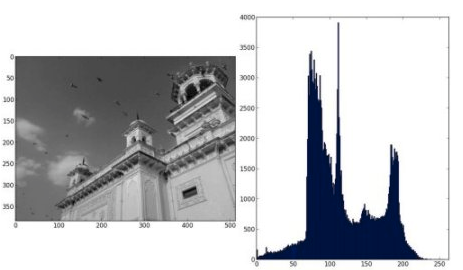import numpy as np
import cv2 as cv
from matplotlib import pyplot as plt
color = ('b','g','r')
for i,col in enumerate(color):
histr = cv.calcHist([img],[i],None,,[0,256])
plt.plot(histr,color = col)
plt.xlim([0,256])
plt.show()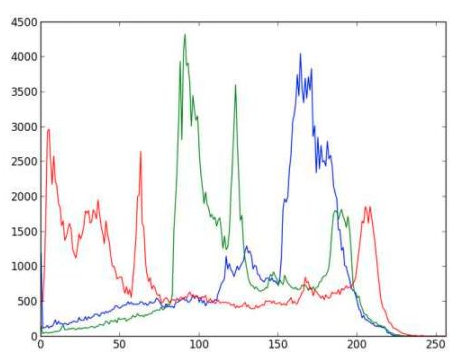• 绘制ROI内的直方图
img = cv.imread('home.jpg',0)
# Check third argument for mask
hist_full = cv.calcHist([img],,None,,[0,256])
plt.subplot(221), plt.imshow(img, 'gray')
plt.xlim([0,256])
plt.show()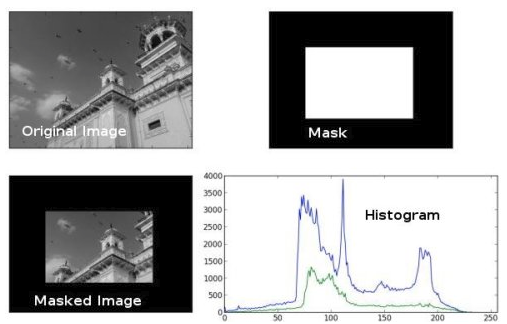## 直方图均衡化

1）变换后图像的灰度级减少，某些细节消失；
2）某些图像，如直方图有高峰，经处理后对比度不自然的过分增强。

这种方法对于背景和前景都太亮或者太暗的图像非常有用，这种方法尤其是可以带来X光图像中更好的骨骼结构显示以及曝光过度或者曝光不足照片中更好的细节。这种方法的一个主要优势是它是一个相当直观的技术并且是可逆操作，如果已知均衡化函数，那么就可以恢复原始的直方图，并且计算量也不大。这种方法的一个缺点是它对处理的数据不加选择，它可能会增加背景杂讯的对比度并且降低有用信号的对比度。

• 函数原型：dst = cv.equalizeHist( src[, dst] )
• src： Source 8-bit single channel image.
• dst： Destination image of the same size and type as src .
import numpy as np
import cv2 as cv
from matplotlib import pyplot as plt
hist,bins = np.histogram(img.flatten(),256,[0,256])
cdf = hist.cumsum()
cdf_normalized = cdf * float(hist.max()) / cdf.max()
plt.plot(cdf_normalized, color = 'b')
plt.hist(img.flatten(),256,[0,256], color = 'r')
plt.xlim([0,256])
plt.legend(('cdf','histogram'), loc = 'upper left')
plt.show()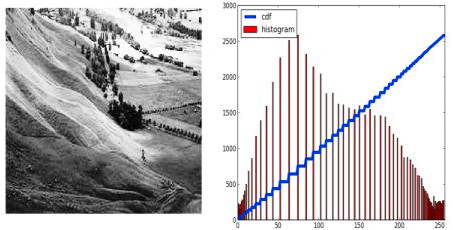• 函数原型：retval = cv.createCLAHE( [, clipLimit[, tileGridSize]] )
• src： Source 8-bit single channel image.
• dst： Destination image of the same size and type as src .
import numpy as np
import cv2 as cv
# create a CLAHE object (Arguments are optional).
clahe = cv.createCLAHE(clipLimit=2.0, tileGridSize=(8,8))
cl1 = clahe.apply(img)
cv.imwrite('clahe_2.jpg',cl1)


## 二维直方图

• channels = [0,1] 因为我们需要同时处理H和S平面（译者注：分别表示H色调和S饱和度）。
• bins = [180,256] H平面最多180个抽屉，S平面最多256个抽屉。
• range = [0,180,0,256] 色调值落在 0 到 180 & 饱和度落在 0 到 256。
import numpy as np
import cv2 as cv
hsv = cv.cvtColor(img,cv.COLOR_BGR2HSV)
hist = cv.calcHist([hsv], [0, 1], None, [180, 256], [0, 180, 0, 256])


Numpy里的2D直方图
Numpy也为此提供了一个特定的函数：np.histogram2d()。

import numpy as np
import cv2 as cv
from matplotlib import pyplot as plt
hsv = cv.cvtColor(img,cv.COLOR_BGR2HSV)
hist, xbins, ybins = np.histogram2d(h.ravel(),s.ravel(),[180,256],[[0,180],[0,256]])


import numpy as np
import cv2 as cv
from matplotlib import pyplot as plt
hsv = cv.cvtColor(img,cv.COLOR_BGR2HSV)
hist = cv.calcHist( [hsv], [0, 1], None, [180, 256], [0, 180, 0, 256] )
plt.imshow(hist,interpolation = 'nearest')
plt.show()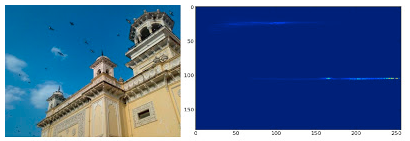## 直方图反向投影

import numpy as np
import cv2 as cvfrom matplotlib import pyplot as plt
#roi is the object or region of object we need to find
hsv = cv.cvtColor(roi,cv.COLOR_BGR2HSV)
#target is the image we search in
hsvt = cv.cvtColor(target,cv.COLOR_BGR2HSV)
# Find the histograms using calcHist. Can be done with np.histogram2d also
M = cv.calcHist([hsv],[0, 1], None, [180, 256], [0, 180, 0, 256] )
I = cv.calcHist([hsvt],[0, 1], None, [180, 256], [0, 180, 0, 256] )


## OpenCV里的反映射

OpenCV 提供了一个内置函数 cv.calcBackProject()。它的参数几乎和 cv.calcHist() 函数一样。其中一个参数是直方图，也就是物体的直方图我们必须找到它。并且，这个物体的直方图参数应该在传入这个反映射函数之前被标准化。它返回的是(那张表示了每个像素点属于我们感兴趣物体的)概率的图像。然后我们用圆盘内核做卷积，应用阈值。以下是我的代码和运行结果：

import numpy as np
import cv2 as cv
hsv = cv.cvtColor(roi,cv.COLOR_BGR2HSV)
hsvt = cv.cvtColor(target,cv.COLOR_BGR2HSV)
# calculating object histogram
roihist = cv.calcHist([hsv],[0, 1], None, [180, 256], [0, 180, 0, 256] )
# normalize histogram and apply backprojection
cv.normalize(roihist,roihist,0,255,cv.NORM_MINMAX)
dst = cv.calcBackProject([hsvt],[0,1],roihist,[0,180,0,256],1)
# Now convolute with circular disc
disc = cv.getStructuringElement(cv.MORPH_ELLIPSE,(5,5))
cv.filter2D(dst,-1,disc,dst)
# threshold and binary AND
ret,thresh = cv.threshold(dst,50,255,0)
thresh = cv.merge((thresh,thresh,thresh))
res = cv.bitwise_and(target,thresh)
res = np.vstack((target,thresh,res))
cv.imwrite('res.jpg',res)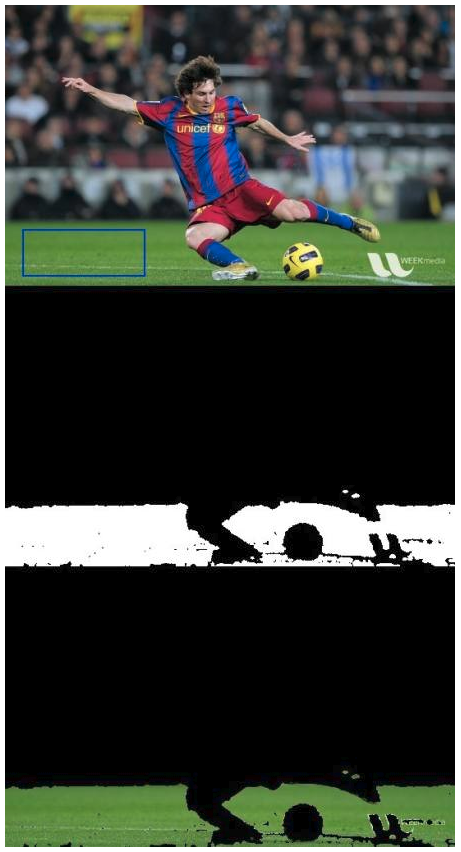# 图像模板匹配（Template Matching）

（1）从输入图像的左上角(0,0)开始，切割一块(0,0)至(10,10)的临时图像；
（2）用临时图像和模板图像进行对比，对比结果记为c；
（3）对比结果c，就是结果图像(0,0)处的像素值；
（4）切割输入图像从(0,1)至(10,11)的临时图像，对比，并记录到结果图像；
（5）重复（1）～（4）步直到输入图像的右下角。

## 单目标模板匹配

• 函数原型：result = cv.matchTemplate( image, templ, method[, result[, mask]] )
• image：待匹配的图像，必须是 8-bit 或者 32-bit 浮点数
• templ： 模板图像，必须小于搜索图像，数据类型与image一致
• result： 模板匹配函数cvMatchTemplate依次计算模板与待测图片的重叠区域的相似度，并将结果存入映射图像result当中，也就是说result图像中的每一个点的值代表了一次相似度比较结果。结果是单通道 32-bit 浮点数。如果image图像大小是W×H，模板图像大小是w×h，则result图像大小是(W−w+1)×(H−h+1)
• method：模板匹配方法。
• CV_TM_SQDIFF 平方差匹配法：该方法采用平方差来进行匹配；最好的匹配值为0；匹配越差，匹配值越大。
• CV_TM_CCORR 相关匹配法：该方法采用乘法操作；数值越大表明匹配程度越好。
• CV_TM_CCOEFF 相关系数匹配法：1表示完美的匹配；-1表示最差的匹配。
• CV_TM_SQDIFF_NORMED 归一化平方差匹配法
• CV_TM_CCORR_NORMED 归一化相关匹配法
• CV_TM_CCOEFF_NORMED 归一化相关系数匹配法
函数cv.minMaxLoc说明
• 函数原型：minVal, maxVal, minLoc, maxLoc = cv.minMaxLoc( src[, mask] )
• src： 输入单通道图像
• minVal：比对结果中的最小值
• maxVal：比对结果中的最大值
• minLoc：比对结果中的最小值位置坐标
• maxLoc：比对结果中的最大值位置坐标
import cv2 as cv
import numpy as np
from matplotlib import pyplot as plt
img2 = img.copy()
w, h = template.shape[::-1]
# All the 6 methods for comparison in a list
methods = ['cv.TM_CCOEFF', 'cv.TM_CCOEFF_NORMED', 'cv.TM_CCORR',
'cv.TM_CCORR_NORMED', 'cv.TM_SQDIFF', 'cv.TM_SQDIFF_NORMED']
for meth in methods:
img = img2.copy()
method = eval(meth)
# Apply template Matching
res = cv.matchTemplate(img,template,method)
min_val, max_val, min_loc, max_loc = cv.minMaxLoc(res)
# If the method is TM_SQDIFF or TM_SQDIFF_NORMED, take minimum
if method in [cv.TM_SQDIFF, cv.TM_SQDIFF_NORMED]:
top_left = min_loc
else:
top_left = max_loc
bottom_right = (top_left + w, top_left + h)
cv.rectangle(img,top_left, bottom_right, 255, 2)
plt.subplot(121),plt.imshow(res,cmap = 'gray')
plt.title('Matching Result'), plt.xticks([]), plt.yticks([])
plt.subplot(122),plt.imshow(img,cmap = 'gray')
plt.title('Detected Point'), plt.xticks([]), plt.yticks([])
plt.suptitle(meth)
plt.show()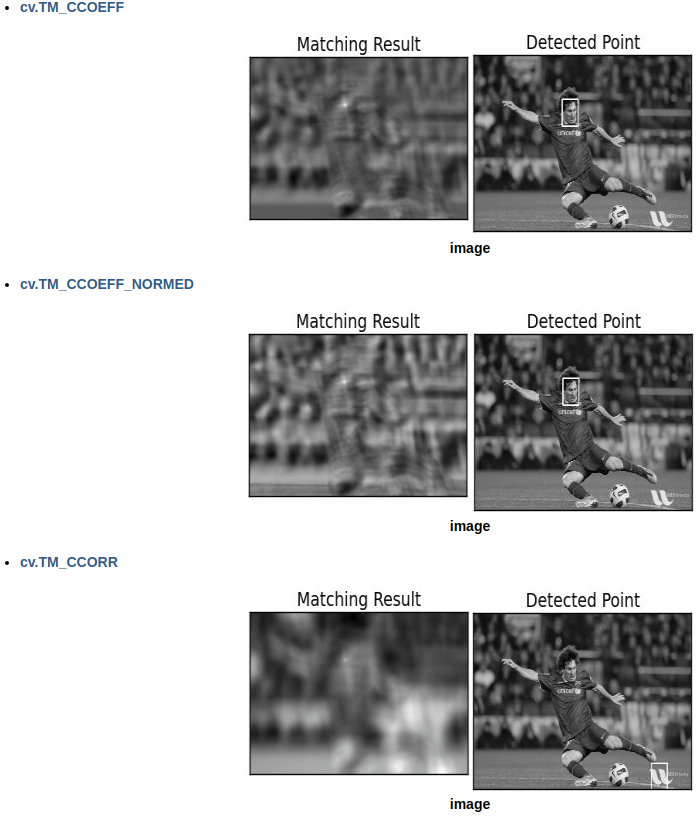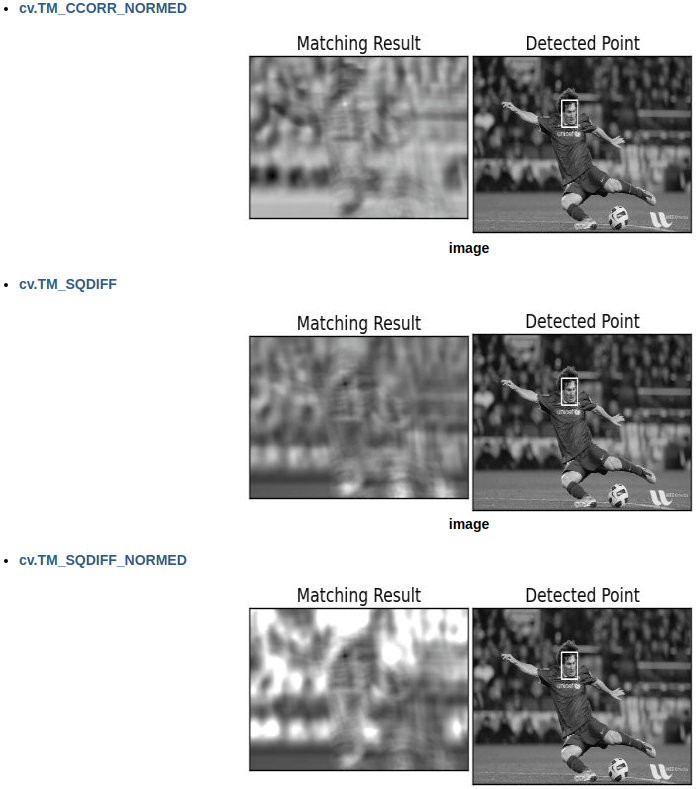## 多目标模板匹配

import cv2 as cv
import numpy as np
from matplotlib import pyplot as plt
img_gray = cv.cvtColor(img_rgb, cv.COLOR_BGR2GRAY)
w, h = template.shape[::-1]
res = cv.matchTemplate(img_gray,template,cv.TM_CCOEFF_NORMED)
threshold = 0.8
loc = np.where( res >= threshold)
for pt in zip(*loc[::-1]):
cv.rectangle(img_rgb, pt, (pt + w, pt + h), (0,0,255), 2)
cv.imwrite('res.png',img_rgb)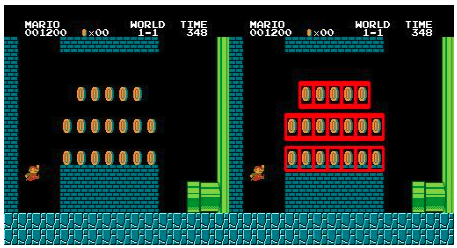# 霍夫直线检测（Hough Line Transform）

• 函数原型： lines = cv.HoughLines( image, rho, theta, threshold[, lines[, srn[, stn[, min_theta[, max_theta]]]]] )
• image：输入图像，要求是单通道的二值图像，输入图像可能被此函数修改
• lines：输出直线向量，两个元素的向量（ρ，θ）代表一条直线，ρ是从原点（图像的左上角）的距离，θ是直线的角度（单位是弧度），0表示垂直线，π/2表示水平线
• rho：像素相关单位的距离加器
• theta：角度分辨率
• threshold：阈值参数，如果相应的累计值大于 threshold， 则函数返回这条线段
• srn：多尺度霍夫直线变换rto参数的除数，它导致累加精度为：rho/srn 。
• stn： 多尺度霍夫直线变换 theta参数的除数
• 如果srn和stn同时为0，就表示使用经典的霍夫变换；否则这两个参数应该都为正数。
• min_theta：检测直线的最小角度θ，取值范围是：0 and max_theta.
• max_theta：检测直线的最大角度θ，取值范围是： min_theta and CV_PI.
import cv2 as cv
import numpy as np
gray = cv.cvtColor(img,cv.COLOR_BGR2GRAY)
edges = cv.Canny(gray,50,150,apertureSize = 3)
lines = cv.HoughLines(edges,1,np.pi/180,200)
for line in lines:
rho,theta = line
a = np.cos(theta)
b = np.sin(theta)
x0 = a*rho
y0 = b*rho
x1 = int(x0 + 1000*(-b))
y1 = int(y0 + 1000*(a))
x2 = int(x0 - 1000*(-b))
y2 = int(y0 - 1000*(a))
cv.line(img,(x1,y1),(x2,y2),(0,0,255),2)
cv.imwrite('houghlines3.jpg',img)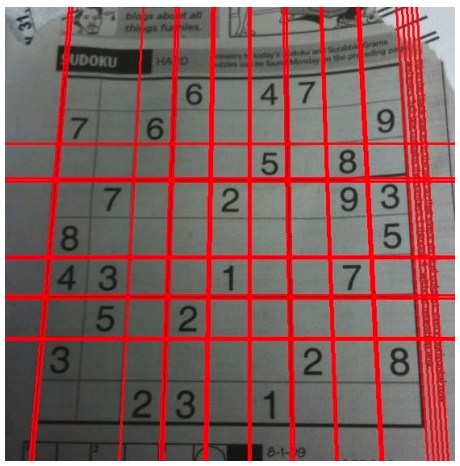• 函数原型： lines = cv.HoughLinesP( image, rho, theta, threshold[, lines[, minLineLength[, maxLineGap]]] )
• image： 必须是二值图像，推荐使用canny边缘检测的结果图像；
• rho: 线段以像素为单位的距离精度，double类型的，推荐用1.0
• theta： 线段以弧度为单位的角度精度，推荐用numpy.pi/180
• threshod: 累加平面的阈值参数，int类型，超过设定阈值才被检测出线段，值越大，基本上意味着检出的线段越长，检出的线段个数越少。根据情况推荐先用100试试
• lines：这个参数的意义未知，发现不同的lines对结果没影响，但是不要忽略了它的存在
• minLineLength：线段以像素为单位的最小长度，小于此值线段不会放入到lines集合
• maxLineGap：同一方向上两条线段判定为一条线段的最大允许间隔，超过了设定值，则为两条线段，值越大，允许线段上的断裂越大。
import cv2 as cv
import numpy as np
gray = cv.cvtColor(img,cv.COLOR_BGR2GRAY)
edges = cv.Canny(gray,50,150,apertureSize = 3)
lines = cv.HoughLinesP(edges,1,np.pi/180,100,minLineLength=100,maxLineGap=10)
for line in lines:
x1,y1,x2,y2 = line
cv.line(img,(x1,y1),(x2,y2),(0,255,0),2)
cv.imwrite('houghlines5.jpg',img)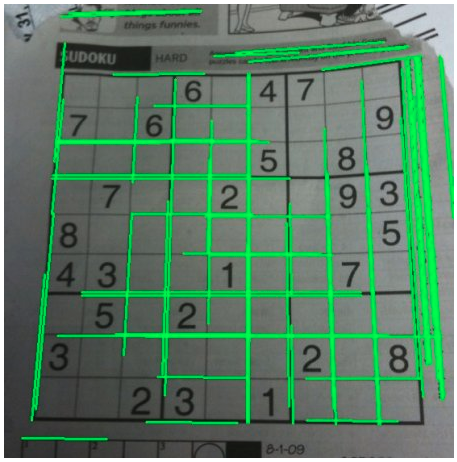# 霍夫圆检测（Hough Circle Transform）

• 函数原型：circles = cv.HoughCircles( image, method, dp, minDist[, circles[, param1[, param2[, minRadius[, maxRadius]]]]] )
• image：8-bit, 单通道灰度图像
• circles：输出圆向量，每个向量包括三个浮点型的元素——圆心横坐标，圆心纵坐标和圆半径
• dp：霍夫空间的分辨率，dp=1时表示霍夫空间与输入图像空间的大小一致，dp=2时霍夫空间是输入图像空间的一半，以此类推，dp的值不能比1小
• minDist：圆心之间的最小距离，如果检测到的两个圆心之间距离小于该值，则认为它们是同一个圆心，取值过小会造成邻近圆检测为一个圆，取值过大回造成一些圆检测丢失。
• param1：Canny的边缘阀值上限，下限被置为上限的一半
import numpy as np
import cv2 as cv
img = cv.medianBlur(img,5)
cimg = cv.cvtColor(img,cv.COLOR_GRAY2BGR)
circles = np.uint16(np.around(circles))
for i in circles[0,:]:
# draw the outer circle
cv.circle(cimg,(i,i),i,(0,255,0),2)
# draw the center of the circle
cv.circle(cimg,(i,i),2,(0,0,255),3)
cv.imshow('detected circles',cimg)
cv.waitKey(0)
cv.destroyAllWindows()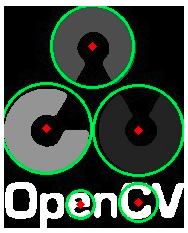# 分水岭算法的图像分割（Image Segmentation with Watershed Algorithm）

• 函数原型：markers = cv.watershed( image, markers )
• image：Input 8-bit 3-channel image.
• markers：Input/output 32-bit single-channel image (map) of markers. It should have the same size as image .
import numpy as np
import cv2 as cv
from matplotlib import pyplot as plt
gray = cv.cvtColor(img,cv.COLOR_BGR2GRAY)
ret, thresh = cv.threshold(gray,0,255,cv.THRESH_BINARY_INV+cv.THRESH_OTSU)
# noise removal
kernel = np.ones((3,3),np.uint8)
opening = cv.morphologyEx(thresh,cv.MORPH_OPEN,kernel, iterations = 2)
# sure background area
sure_bg = cv.dilate(opening,kernel,iterations=3)
# Finding sure foreground area
dist_transform = cv.distanceTransform(opening,cv.DIST_L2,5)
ret, sure_fg = cv.threshold(dist_transform,0.7*dist_transform.max(),255,0)
# Finding unknown region
sure_fg = np.uint8(sure_fg)
unknown = cv.subtract(sure_bg,sure_fg)
# Marker labelling
ret, markers = cv.connectedComponents(sure_fg)
# Add one to all labels so that sure background is not 0, but 1
markers = markers+1
# Now, mark the region of unknown with zero
markers[unknown==255] = 0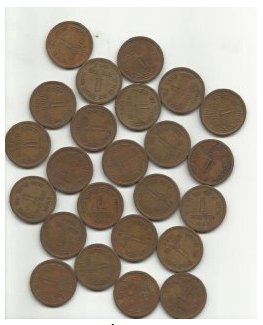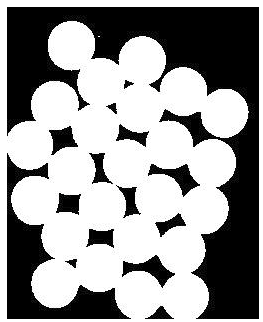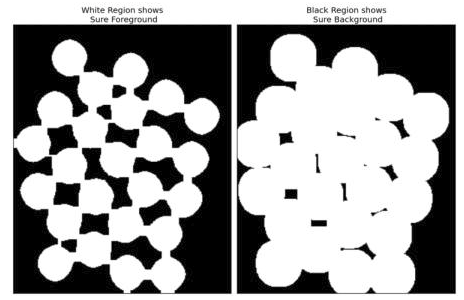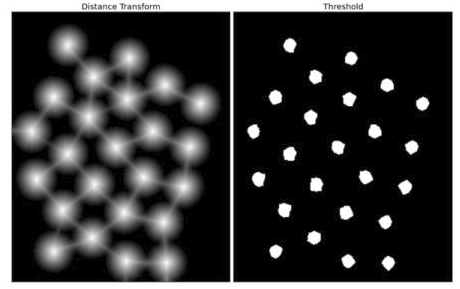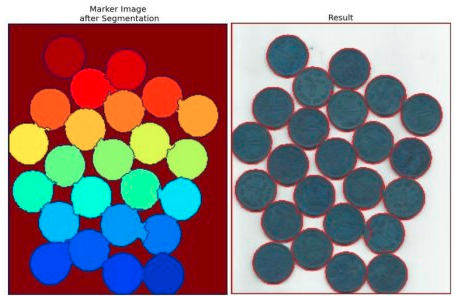# 基于GrabCut算法的交互式前景提取（Interactive Foreground Extraction using GrabCut Algorithm）

GrabCut算法我解释不来，我只能说明一下函数的用法。

• 函数原型：mask, bgdModel, fgdModel = cv.grabCut( img, mask, rect, bgdModel, fgdModel, iterCount[, mode] )
• img：待分割的源图像，必须是8位3通道（CV_8UC3）图像，在处理的过程中不会被修改
GCD_BGD（=0），背景；
GCD_FGD（=1），前景；
GCD_PR_BGD（=2），可能的背景；
GCD_PR_FGD（=3），可能的前景。
如果没有手工标记GCD_BGD或者GCD_FGD，那么结果只会有GCD_PR_BGD或GCD_PR_FGD
• rect：用于限定需要进行分割的图像范围，只有该矩形窗口内的图像部分才被处理，此参数仅在mode==GC_INIT_WITH_RECT有效
• bgdModel：前景模型，如果为null，函数内部会自动创建一个fgdModel；fgdModel必须是单通道浮点型（CV_32FC1）图像，且行数只能为1，列数只能为13x5
• fgdModel：前景模型，如果为null，函数内部会自动创建一个fgdModel；fgdModel必须是单通道浮点型（CV_32FC1）图像，且行数只能为1，列数只能为13x5
• iterCount：迭代次数，必须大于0；返回结果前结果可能会被优化，如果 mode == GC_INIT_WITH_MASK or mode==GC_EVAL .
• mode：用于指示grabCut函数进行什么操作，可选的值有：
GC_INIT_WITH_RECT（=0），用矩形窗初始化GrabCut；
GC_EVAL（=2），执行分割
import numpy as np
import cv2 as cv
from matplotlib import pyplot as plt
bgdModel = np.zeros((1,65),np.float64)
fgdModel = np.zeros((1,65),np.float64)
rect = (50,50,450,290)
plt.imshow(img),plt.colorbar(),plt.show()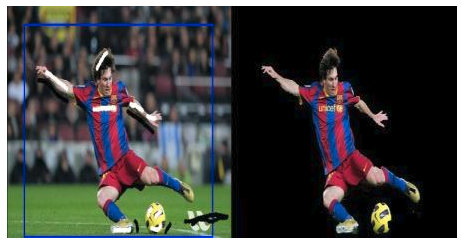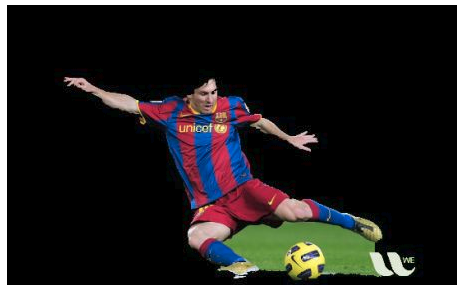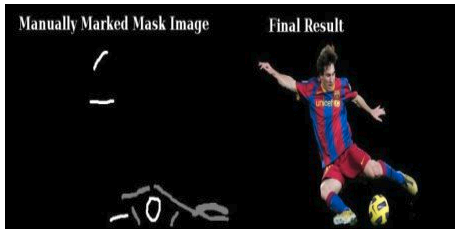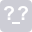Python+OpenCV计算机视觉
12-28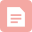博客
Opencv 中类似matlab的subplot功能
05-032646博客
Python-Opencv学习总结：直方图绘制、掩膜、subplot函数、直方图均衡化函数equalizeHist、
12-16562博客

02-064815博客
OpenCV一个窗口画多个图
03-211750博客

02-18701博客
Opencv中的cv2.calcHist()函数的作用及返回值
01-073294博客
【翻译：OpenCV-Python教程】OpenCV里的直方图
12-11554博客
OpenCV图像直方图
07-08241博客

05-05196博客
python数字图像处理9：直方图与均衡化

01-253万+博客
OpenCV-Python】20.OpenCV的直方图均衡化
12-231493博客
flatten函数
02-15791博客
【学习笔记】OpenCv图像处理基础

03-144672博客
OpenCV 多幅图像 同一个窗口 显示 代码优化版
03-219849博客
pyplot模块介绍opencvpython实现
01-19204博客
Python-OpenCV中的cv2.inpaint()函数
03-282224博客
Python+OpenCV学习18---双目视觉深度信息恢复
09-209854博客
flatten()函数用法
09-014012

### “相关推荐”对你有帮助么？

•非常没帮助
•没帮助
•一般
•有帮助
•非常有帮助luke-skyworker

¥2 ¥4 ¥6 ¥10 ¥20余额支付 (余额：-- )扫码支付获取中扫码支付点击重新获取扫码支付1.余额是钱包充值的虚拟货币，按照1:1的比例进行支付金额的抵扣。
2.余额无法直接购买下载，可以购买VIP、C币套餐、付费专栏及课程。余额充值